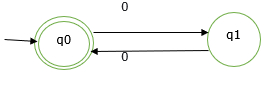# Explain the concept of Regular expression.

Regular expression is the language which is used to describe the language and is accepted by the finite automata. Regular expressions are the most effective way to represent any language.

Regular language is the language which is accepted by some regular expression.

Regular sets are sets which are accepted by finite automata.

## Example

L={ ε,00,0000,000000,……..}

This set represents the set of even numbers of zero’s.

We can represent this set by finite automata as follows

M = ({q0, q1}, {0}, δ, q0, q0)The given set L is said to be a regular set because it is represented by finite automata.

## Regular expressions

Let Σ be an alphabet which denotes the input set.

The regular expression over Σ can be defined as follows −

• Φ is a regular expression which denotes the empty set.
• ε is a regular expression and denotes the set { ε} and it is called a null string.
• For each ‘a’ in Σ ‘a’ is a regular expression and denotes the set {a}.
• If r and s regular expressions denoting the language.
• L1 and l2 respectively then,
• r+s is equivalent to L1 U L2 union
• rs is equivalent to L1L2 concatenation
• r* is equivalent to L1* closure

The r* is known as Kleen closure or closure which indicates occurrence of r for an infinite number of times.

## Example

If Σ ={a} and we have regular expression

R=a*

Then R is a set denoted by R={ ε,a,aa,aaa,aaaa,………}

That is R includes any number of a’s as well as empty string which indicates zero number of a’s appearing, denoted by ε character.